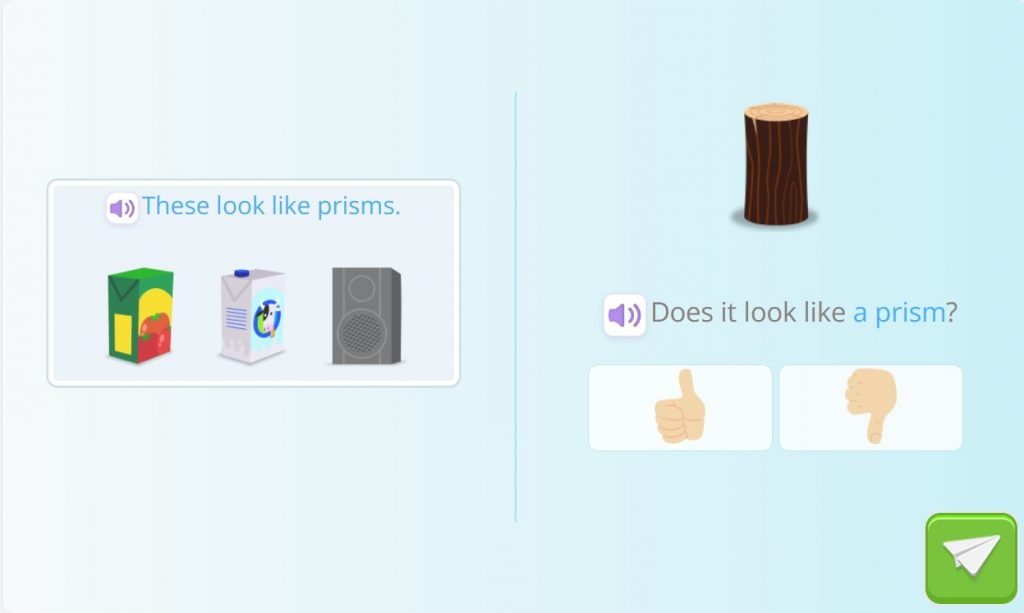Smartick is a fun way to learn math!Jun27

# What is a Prism? Solve these Exercises to Find Out

Welcome to this post about prisms! Have you ever wondered what is a prism? Look no further! In this guide, we will explain everything you need to know about prisms.

Index

## Is this figure a prism?

Before we dive into the fascinating world of these three-dimensional geometric figures, we are going to test your skills with an exercise. We’ll show you some figures and your task will be to determine whether they are prisms or not and then, figure out what is a prism. Get ready for the challenge!

• Exercise 1: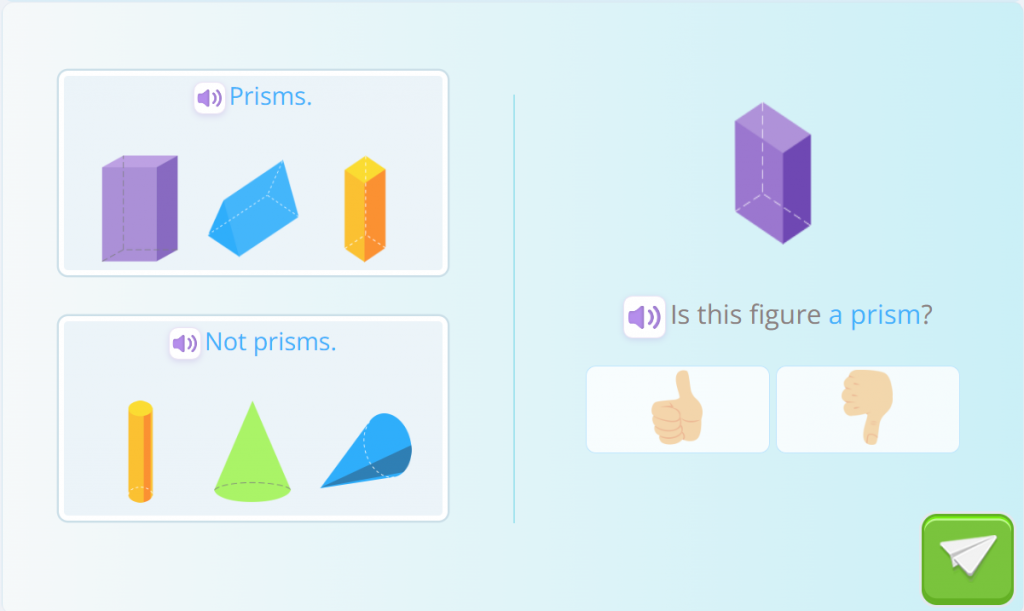• Exercise 2: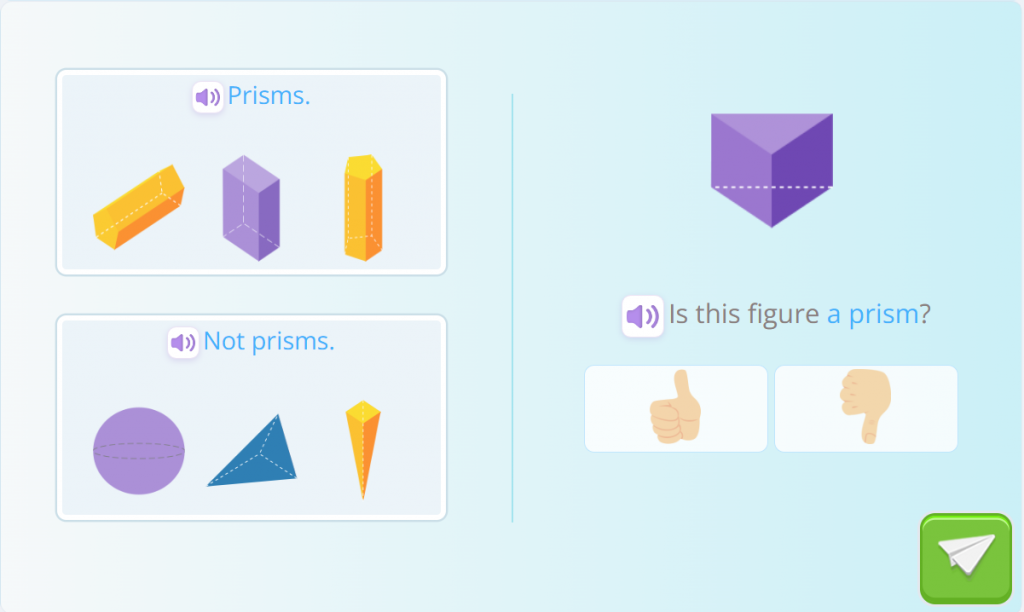• Exercise 3: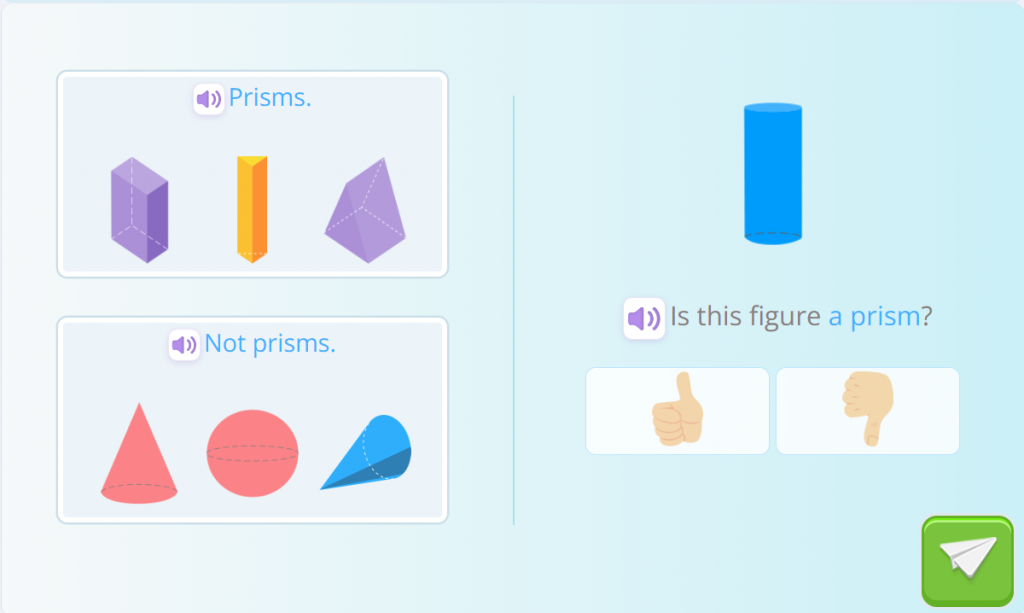Let’s learn what is a prism and, at the end, check to see if you got the answers right.

## What is a prism?

A prism is a three-dimensional geometric figure that has two parallel and equal bases, which are polygons. These bases are joined by lateral faces that are also polygons. Prisms exist in various shapes and sizes, which makes them very interesting objects to learn about.To understand it better, imagine you are holding a rectangular box in your hands. This box is a concrete example of a prism. Its base is a rectangle, and the lateral faces are also rectangles. However, prisms can have bases in the form of triangles, squares and even more complex polygons.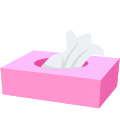## How do we classify prisms?

Prisms can be classified according to the number of sides of their bases. For example, if a prism has a triangular base, it is called a triangular prism. On the other hand, if it has a square base, it is known as a quadrangular prism. If the bases are parallelograms and the angles are right angles, it is called a rectangular prism. And remember, a prism is still a prism even if  “falls over” and its bases are not at the bottom and top of the figure. Check out these examples of prisms and their names!

… and many more!

A special case of a prism is a cube, which equal faces; all its faces are squares!

## What else do I need to know?

When studying prisms, it is also important to know some additional terms. For example, the height of a prism is the perpendicular distance between the bases, and the edge is the segment that joins two consecutive vertices of one base with the corresponding vertices of the other base.

## Area and volume of prisms

To get the total area of the prism, think carefully about what a prism is and… you will certainly figure out how to calculate the area! A prism can be developed like this:We can simply calculate the area of the bases and the area of the lateral faces and then add it all up. But if you want, to know the exact formula, we have another post you can visit.

But that’s not all. You can also calculate the volume of a prism by multiplying the area of the base by the height of the prism.

The formula is:

Volume = Base area × height.

This way, you will be able to determine how much space it can hold inside.

## Examples of prisms in real life

Prisms are present in numerous everyday objects. Prisms are used in a variety of areas, from architecture to engineering. Architects can use prisms to design and construct impressive buildings, while engineers can use them to create strong and efficient structures.

You can find them in buildings, food packaging, toy boxes and many other places. We encourage you to look around and try to identify the prisms you find – don’t be surprised if you start seeing them everywhere!## Solved exercises

Now that we have learned and reviewed a little more about what is a prism, we are ready to check the exercises from before!

• Exercise 1: Yes, this figure is a prism. It has two equal, parallel rectangular bases and lateral faces that are also rectangles. It is a rectangular prism.
• Exercise 2: Yes, this figure is also a prism. It has two parallel and equal triangular bases and lateral faces that are rectangles. It is a triangular prism.
• Exercise 3: No, it is not a prism. It has two parallel and equal bases, but they are circular, not polygonal. Although we have not seen it in this posy, this figure is a cylinder.

With this guide on prisms and the challenge of identifying these figures, I hope you have enjoyed learning and are ready to delve even deeper into the study of these fascinating geometric figures. Check out Smartick to continue learning about prisms and many other topics.

And finally, why don’t you try to solve this exercise with real life examples. Tell me, does it look like a prism?